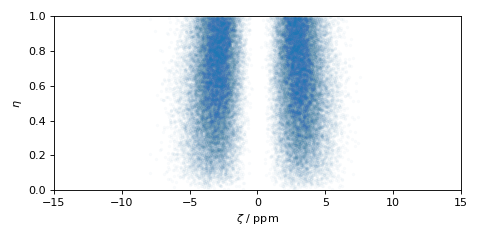# Czjzek distribution¶

The Czjzek distribution models random variations of a second-rank traceless symmetric tensors about zero, i.e., a tensor with zeta of zero. See Czjzek distribution and references within for a brief description of the model.

## Czjzek distribution of symmetric shielding tensors¶

To generate a Czjzek distribution, use the CzjzekDistribution class as follows.

>>> from mrsimulator.models import CzjzekDistribution
>>> cz_model = CzjzekDistribution(sigma=0.8)


The CzjzekDistribution class accepts a single argument, sigma, which is the standard deviation of the second-rank traceless symmetric tensor parameters. In the above example, we create cz_model as an instance of the CzjzekDistribution class with $$\sigma=0.8$$.

Note, cz_model is only a class instance of the Czjzek distribution. You can either draw random points from this distribution or generate a probability distribution function. Let’s first draw points from this distribution, using the rvs() method of the instance.

>>> zeta_dist, eta_dist = cz_model.rvs(size=50000)


In the above example, we draw size=50000 random points of the distribution. The output zeta_dist and eta_dist hold the tensor parameter coordinates of the points, defined in the Haeberlen convention. The scatter plot of these coordinates is shown below.

>>> import matplotlib.pyplot as plt
>>> plt.scatter(zeta_dist, eta_dist, s=4, alpha=0.02)
>>> plt.xlabel('$\zeta$ / ppm')
>>> plt.ylabel('$\eta$')
>>> plt.xlim(-15, 15)
>>> plt.ylim(0, 1)
>>> plt.tight_layout()
>>> plt.show()


(png, hires.png, pdf)## Czjzek distribution of symmetric quadrupolar tensors¶

The Czjzek distribution of symmetric quadrupolar tensors follows a similar setup as the Czjzek distribution of symmetric shielding tensors, except we assign the outputs to Cq and $$\eta_q$$. In the following example, we generate the probability distribution function using the pdf() method.

>>> import numpy as np
>>> Cq_range = np.arange(100)*0.3 - 15 # pre-defined Cq range in MHz.
>>> eta_range = np.arange(21)/20  # pre-defined eta range.
>>> Cq, eta, amp = cz_model.pdf(pos=[Cq_range, eta_range])


To generate a probability distribution, we need to define a grid system over which the distribution probabilities will be evaluated. We do so by defining the range of coordinates along the two dimensions. In the above example, Cq_range and eta_range are the range of $$\text{Cq}$$ and $$\eta_q$$ coordinates, which is then given as the argument to the pdf() method. The output Cq, eta, and amp hold the two coordinates and amplitude, respectively.

The plot of the Czjzek probability distribution is shown below.

>>> import matplotlib.pyplot as plt
>>> plt.contourf(Cq, eta, amp, levels=10)
>>> plt.xlabel('$C_q$ / MHz')
>>> plt.ylabel('$\eta$')
>>> plt.tight_layout()
>>> plt.show()


(png, hires.png, pdf)Note

The pdf method of the instance generates the probability distribution function by first drawing random points from the distribution and then binning it onto a pre-defined grid.